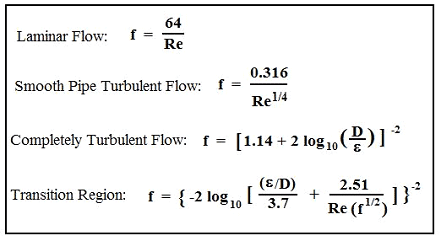Pipe Flow Rate and Velocity Calculator

Fluid Flow Design and Engineering

Calculator will determine Pipe Flow Rate and Velocity required based on Head Loss, hL, and Frictional Pressure Drop, DPf, for given flow rate, Q, pipe diam., D, pipe length, L, pipe roughness, e, and fluid properties, r & m.

Moody Friction Factor EquationPipe Roughness ValuesAll calculators require a Premium Membership

NOTE: This is an iterative calculation, because an assumed value of Q is used to start the calculations. If the final calculated value of Q is different from the assumed value, then the assumed value of Q should be replaced with the calculated value of Q, leading to a new calculated value for Q. This should be repeated as many times as necessary to get the calculated value for Q to be the same as the assumed value. This iteration typically converges rather rapidly.

This spreadsheet shows only the final assumed and calculated values of Q (when they are equal). If the initial assumed value of Q was 1.5 cfs, then subsequent calculated and assumed values for Q, leading to the final solution are as follows:

 Assumed Q, cfs Calculated Q, cfs 1.5 0.85 0.85 0.83 0.83 0.83

Related:Membership Register | LoginHomeEngineering Book StoreEngineering ForumExcel App. DownloadsOnline Books & ManualsEngineering NewsEngineering VideosEngineering CalculatorsEngineering ToolboxGD&T Training Geometric Dimensioning TolerancingDFM DFA TrainingTraining Online EngineeringAdvertising CenterCopyright Notice